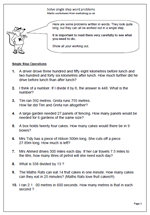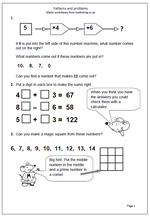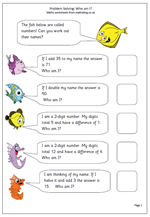## Year 5 maths worksheet: solve single step word problemsThis page contains a set of ten problems written in words. They look quite long, but they can all be worked out using a single step, using one of the operations: addition, subtraction, multiplication and division. Because they only require one step they are easier than questions which involve two or steps, but each calculation is quite tricky, with several needing a good knowledge of written methods to be able to do them.

It is important that all working out is shown for several reasons, including to show that a calculator was not used and to be able to spot where errors occur. Wrong answers are an excellent step towards new learning!

It is also interesting to see how children approach the questions. One or two questions in particular may cause problems. Question 6, involves subtracting of decimals and can be laid out in the standard format but could also be answered by adding on: e.g.

27.89 + 0.11 = 28

28 + 72 = 100

100 + 200 = 300

answer = 0.11 + 72 + 200 = 272.11

Solve single step word problems

## Patterns and problems in Year 4There are many different ways of presenting number problems to children, other than just the traditional ‘sums’ style approach. This page shows three very different approaches to questions that many children will find quite hard.

The first is in the style of a function machine, i.e. a number is put into the machine, various operations are carried out and a number will come out at the end. For this function machine the operations are: x 4 and + 6. The hardest part of this is the last question where the output is given and the input has to be worked out and the machine can be reversed by subtracting 6 and dividing by 4 to get the answer, although many children will use trial and error/improvement methods.

The number sentences in the second question are quite straightforward apart from the fact that both tens and units digits are missing. it is easiest to take one part at a time e.g. find the missing unit and then the missing ten. Watch out for the subtraction!

By year 5 children should be used to magic squares and not find this too tricky, especially with the clue to help.

This page can be found in our year 4, Using and Applying Maths category.

Patterns and problems

## Problem Solving Worksheet for Year 4There are only 5 questions on this page but some children will find it quite difficult. The main reason for this is that they will have to work back from the answer to find the starting number. This will often mean reversing the operations, subtracting where it says add, halving where it says double etc.

This is a god test of children’s knowledge of the relationships between addition and subtraction, doubling and halving and multiplication and division.

Two of the harder questions come at the end, because they involve carrying out two processes to reach the answer. For example:

‘I am a 2-digit number. My digits total 10 and have a difference of 2. Who am I?’

This involves finding 2 digits which have a total of 10 (2 and 8, 3 and 7 etc) and then checking that the difference between the two digits is 2.

6 and 4 will solve the problem so there are two possible answers: 46 or 64.

Solve problems: Who am I?